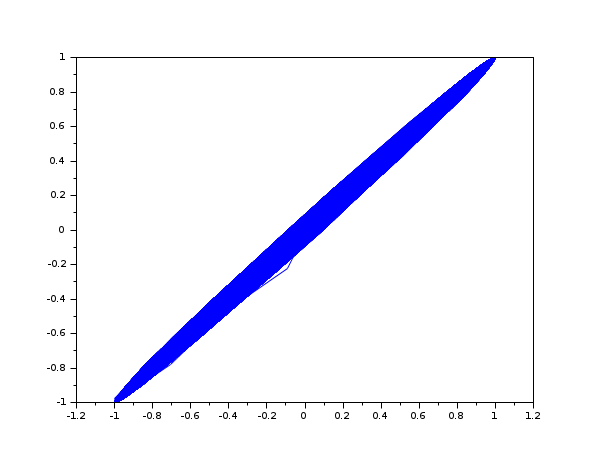Scilab Home page | Wiki | Bug tracker | Forge | Mailing list archives | ATOMS | File exchange
Scilab 5.4.1
Change language to: English - Português - 日本語 - Русский

See the recommended documentation of this function

# intdec

Changes sampling rate of a signal

### Calling Sequence

`[y]=intdec(x,lom)`

### Arguments

x

input sampled signal

lom

For a 1D signal this is a scalar which gives the rate change. For a 2D signal this is a 2-Vector of sampling rate changes `lom`=(col rate change,row rate change)

y

Output sampled signal

### Description

Changes the sampling rate of a 1D or 2D signal by the rates in lom

### Examples

```Fs1 = 10000;
Fs2 = 14000;
t1 = 0:1/Fs1:10;
t2 = 0:1/Fs2:10;
F0 = 500;
u1 = sin(2*%pi*F0*t1);
u2 = sin(2*%pi*F0*t2);
u2b = intdec(u1, Fs2/Fs1)
plot(u2b, u2);```